# Colleges with the highest SAT scores in North Carolina

Top 10 colleges in North Carolina with the highest SAT scores
Looking for the colleges with the highest SAT scores in North Carolina? Well you're in luck! We've compiled a national college database and have created a list of the top 10 universities with the highest SAT scores in North Carolina. These are the schools whose applicants had the highest average SAT scores in North Carolina. And, since these tests are meant to determine academic prowess, they are arguably the schools with the most academically proficient students. You could even say these are the best colleges in North Carolina. We also include each college's ACT scores and acceptance rate so that you can see where you would have the easiest or hardest time getting in. Read on to find out more.

## Duke University SAT scores

The average SAT score for Duke University is 1510.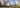The average SAT score of 1510 breaks down into:

• SAT math: 770

The average ACT score for Duke University is 34 and their acceptance rate is 8.9%.

## University of North Carolina SAT scores

The average SAT score for University of North Carolina is 1395.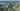The average SAT score of 1395 breaks down into:

• SAT math: 705

The average ACT score for University of North Carolina is 30 and their acceptance rate is 22.6%.

## University of North Carolina at Chapel Hill SAT scores

The average SAT score for University of North Carolina at Chapel Hill is 1395.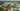The average SAT score of 1395 breaks down into:

• SAT math: 705

The average ACT score for University of North Carolina at Chapel Hill is 30 and their acceptance rate is 22.6%.

## Wake Forest University SAT scores

The average SAT score for Wake Forest University is 1390.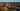The average SAT score of 1390 breaks down into:

• SAT math: 710

The average ACT score for Wake Forest University is 31 and their acceptance rate is 29.4%.

## Davidson College SAT scores

The average SAT score for Davidson College is 1372.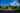The average SAT score of 1372 breaks down into:

• SAT math: 690

The average ACT score for Davidson College is 31 and their acceptance rate is 19.5%.

## North Carolina State University SAT scores

The average SAT score for North Carolina State University is 1335.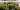The average SAT score of 1335 breaks down into:

• SAT math: 680

The average ACT score for North Carolina State University is 30 and their acceptance rate is 44.9%.

## University of North Carolina Wilmington SAT scores

The average SAT score for University of North Carolina Wilmington is 1260.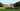The average SAT score of 1260 breaks down into:

• SAT math: 630

The average ACT score for University of North Carolina Wilmington is 25 and their acceptance rate is 61.2%.

## Elon University SAT scores

The average SAT score for Elon University is 1238.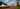The average SAT score of 1238 breaks down into:

• SAT math: 620

The average ACT score for Elon University is 27 and their acceptance rate is 72.1%.

## University of North Carolina Charlotte SAT scores

The average SAT score for University of North Carolina Charlotte is 1195.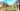The average SAT score of 1195 breaks down into:

• SAT math: 600

The average ACT score for University of North Carolina Charlotte is 24 and their acceptance rate is 67.2%.

## Salem College SAT scores

The average SAT score for Salem College is 1190.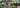The average SAT score of 1190 breaks down into:

• SAT math: 570